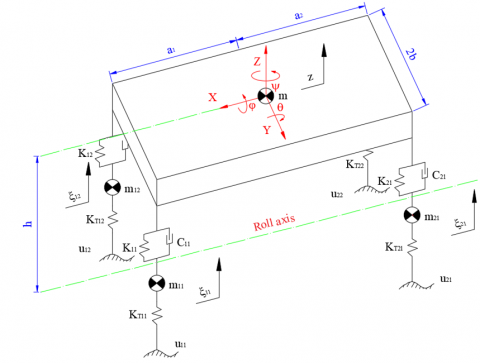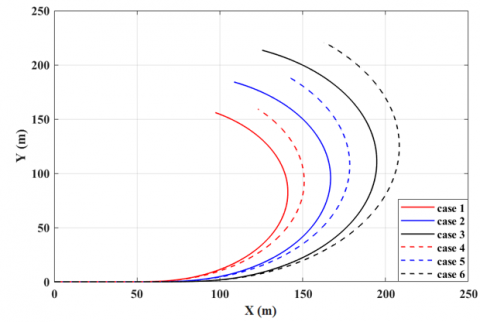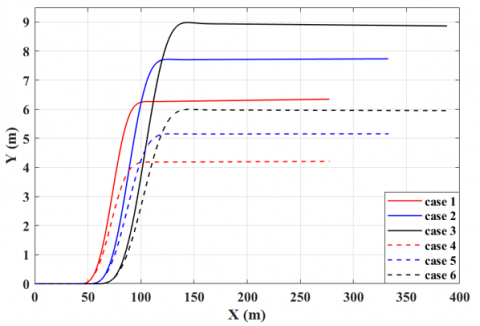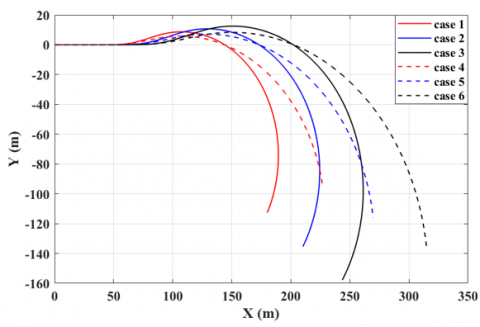# Establishing the Dynamics Model of the Vehicle Using the 4-Wheels Steering Systems

Establishing the Dynamics Model of the Vehicle Using the 4-Wheels Steering Systems
Automotive Engineering Department, Thuyloi University, 175 Tay Son, Dong Da, Hanoi, Vietnam

Corresponding Author Email:
anhngtu@tlu.edu.vn
Page:
436-440
|
DOI:
https://doi.org/10.18280/mmep.070314
18 June 2020
|
Accepted:
22 August 2020
|
Published:
30 September 2020
| Citation

OPEN ACCESS

Abstract:

The safety and stability of the vehicle are some of the most important factors when the vehicle moves on the road. If the driver suddenly steers at high speed, it may cause instability (oversteering, understeering). One of the solutions used to limit this situation is to use a 4-wheels steering system instead of the conventional 2-wheels steering system. This article focuses on establishing the spatial dynamics model of the vehicle and simulating the trajectory of the vehicle in the case of 4-wheels steering and 2-wheels steering in different moving conditions. The results of the study show that when the vehicle was equipped with the 4-wheels steering system, the stability of the vehicle was improved. Therefore, if the vehicles use the 4-wheels steering system, the oversteering and understeering phenomenon can be reduced.

Keywords:

dynamic vehicle, 4-wheels steering, understeering, oversteering

1. Introduction

The automobiles are versatile vehicles commonly used around the world. With the development of technology, engineering, and infrastructure, vehicles can move at very high speeds. However, this has also increased the number of crashes, rollovers, … One of the leading causes of these accidents is instability when changing the direction of the movement of the vehicle. This instability is characterized by the steering system through two phenomena: oversteering and understeering [1, 2].

The oversteering phenomenon occurs mainly when the vehicle turns around at high speed. At this time, the turning radius of the vehicle is quite small. With the sudden appearance of the centrifugal force, the vehicle can be rollover in an extremely small amount of time. To limit this phenomenon, the trajectory of the vehicle (turning radius) needs to be increased.

The understeering phenomenon often occurs when the vehicle changes lanes at high speed. The trajectory of movement (lateral) of the vehicle is quite large. Therefore, the vehicle will be deviated from the lanes and risk hitting other obstacles such as median strips, adjacent vehicles, … To minimize this situation, it is necessary to reduce the trajectory (lateral) of the vehicle when changing lanes at high speed.

Several solutions have been introduced to improve the stability of the vehicle when steering such as equipping the Electronic Stability Program (ESP), Active Suspension (AS), … However, this problem has not been resolved yet. Today, modern vehicles are using the 4-wheels steering system instead of the 2-wheels steering system to improve safety and stability when steering. Actual results show that safety and stability have been significantly improved .

Rehan et al.  demonstrated that the turning radius of the vehicle equipped with the 4-wheels steering system will be smaller than that of the conventional 2-wheels steering system. Singh, A. et al also verified these problems in their study . Spentzas et al.  introduced the dynamics model of the 4-wheels steering that used simple modeling.

Many studies on 4-wheels steering systems to improve stability and minimize the turning radius of the vehicles have been performed [7-9]. Gao et al.  introduced the algorithm to control the 4-wheels steering system by the LQR controller. Ping, X. et al also showed a simulation method of the 4-wheels steering system based on the PID control algorithm . Besides, studies on control and coordination of the 4-wheels electronic steering system have also been conducted [12-24]. In general, the results of these studies show that the 4-wheels steering system helps the vehicles move more safely and stably, limiting the effects of the oversteering and understeering at high speed.

This study focuses on establishing the dynamic model of the vehicle when steering and simulating the trajectory under different steering conditions to show the difference between the 4-wheels steering system and the 2-wheels steering system.

2. Methodology

2.1 Establish the double-track dynamic model

To determine the vehicle's trajectory when steering, the double-track dynamic model was used (Figure 1). The movement of the vehicle is described based on the following 3 equations below.

The equation describing the longitudinal movement of the vehicle:

$M\left[ {{{\dot{v}}}_{x}}-\left( \dot{\alpha }+\dot{\psi } \right){{v}_{y}} \right]=\sum\limits_{i,j=1}^{2}{\left( {{F}_{xij}}cos{{\delta }_{ij}}-{{F}_{yij}}sin{{\delta }_{ij}} \right)-{{F}_{1}}}$          (1)

The equation describing the lateral movement of the vehicle:

$M\left[ {{{\dot{v}}}_{y}}+\left( \dot{\alpha }+\dot{\psi } \right){{v}_{x}} \right]=\sum\limits_{i,j=1}^{2}{\left( {{F}_{xij}}sin{{\delta }_{ij}}+{{F}_{yij}}cos{{\delta }_{ij}} \right)-{{F}_{2}}}$          (2)

The equation describing the rotation of the vehicle around the axis:

{{I}_{Z}}\ddot{\psi }=\sum\limits_{i,j=1}^{2}{\left[ \begin{align} & {{\left( -1 \right)}^{j}}\left( {{F}_{xij}}cos{{\delta }_{ij}}-{{F}_{yij}}sin{{\delta }_{ij}} \right){{t}_{wi}}+{{F}_{i}}{{c}_{i}}+ \\ & {{\left( -1 \right)}^{i+1}}\left( {{F}_{xij}}sin{{\delta }_{ij}}+{{F}_{yij}}cos{{\delta }_{ij}} \right){{a}_{i}}-{{M}_{zij}} \\ \end{align} \right]}           (3)

## 1.pngFigure 1. Double-track dynamics model

The trajectory of the vehicle is determined based on the position of the center of gravity of the vehicle. Therefore, these values need to be calculated.

$X=\int{vcos\left( \alpha +\psi \right)dt}$          (4)

$Y=\int{vsin\left( \alpha +\psi \right)dt}$          (5)

The value of the slip angle a can be calculated according to (6):

$\alpha =arctan\frac{{{v}_{y}}}{{{v}_{x}}}$          (6)

2.2 Determine the forces of the tires

The value of the longitudinal force Fx, lateral force Fy, and moment Mz are the function that depends on the vertical force Fz of the tires.

${{F}_{x}},{{F}_{y}},{{M}_{z}}=f\left( {{F}_{z}} \right)$           (7)

To determine these values, the Pacejka tire model is used in this study .

The longitudinal force of the wheels:

{{F}_{x}}={{D}_{x}}sin\left( {{C}_{x}}arctan\left[ \begin{align} & {{B}_{x}}(1-{{E}_{x}})({{s}_{x}}+{{S}_{hx}})+ \\ & {{E}_{x}}arctan\left\{ {{B}_{x}}({{s}_{x}}+{{S}_{hx}}) \right\} \\ \end{align} \right] \right)+{{S}_{vx}}          (8)

The lateral force of the wheels:

{{F}_{y}}={{D}_{y}}sin\left( {{C}_{y}}arctan\left[ \begin{align} & {{B}_{y}}(1-{{E}_{y}})(\alpha +{{S}_{hy}})+ \\ & {{E}_{y}}arctan\left\{ {{B}_{y}}(\alpha +{{S}_{hy}}) \right\} \\ \end{align} \right] \right)+{{S}_{vy}}          (9)

The moment of the wheels:

{{M}_{z}}={{D}_{z}}sin\left( {{C}_{z}}arctan\left[ \begin{align} & {{B}_{z}}(1-{{E}_{z}})(\alpha +{{S}_{hz}})+ \\ & {{E}_{z}}arctan\left\{ {{B}_{z}}(\alpha +{{S}_{hz}}) \right\} \\ \end{align} \right] \right)+{{S}_{vz}}          (10)

The coefficients B, C, D, E, s, and S are referenced in . The value of the vertical force Fz is calculated based on the spatial dynamics model of the vehicle.

2.3 Determine the vertical force

To calculate the vertical force of the wheels, the spatial dynamics model of the vehicle is established [27, 28].

The equations describe the oscillation of the vehicle with 7 degrees of freedom in space as below (Figure 2):

$m\ddot{z}={{F}_{C11}}+{{F}_{K11}}+{{F}_{C12}}+{{F}_{K12}}+{{F}_{C21}}+{{F}_{K21}}+{{F}_{C22}}+{{F}_{K22}}$          (11)

\begin{align} & \left( {{I}_{x}}+m{{h}^{2}} \right)\ddot{\varphi }=\left( {{F}_{C11}}+{{F}_{K11}}-{{F}_{C12}}-{{F}_{K12}} \right){{t}_{w1}}+ \\ & \left( {{F}_{C21}}+{{F}_{K21}}-{{F}_{C22}}-{{F}_{K22}} \right){{t}_{w2}}+\left( gsin\varphi +{{a}_{y}}cos\varphi \right)mh \\ \end{align}           (12)

\begin{align} & \left( {{I}_{y}}+mh_{1}^{2} \right)\ddot{\theta }=\left( {{F}_{C11}}+{{F}_{K11}}+{{F}_{C12}}+{{F}_{K12}} \right){{a}_{1}}- \\ & \left( {{F}_{C21}}+{{F}_{K21}}+{{F}_{C22}}+{{F}_{K22}} \right){{a}_{2}} \\ \end{align}          (13)

${{m}_{ij}}{{\ddot{\xi }}_{ij}}={{F}_{KTij}}-{{F}_{Cij}}-{{F}_{Kij}}$        $i,j=\overline{1,2}$          (14)

where:

${{F}_{Cij}}={{C}_{ij}}\left[ {{{\dot{\xi }}}_{ij}}-\dot{z}+{{\left( -1 \right)}^{i}}{{b}_{i}}\dot{\varphi } \right]$  $i,j=\overline{1,2}$          (15)

${{F}_{Kij}}={{K}_{ij}}\left[ {{\xi }_{ij}}-z+{{\left( -1 \right)}^{i}}{{b}_{i}}\varphi \right]$  $i,j=\overline{1,2}$           (16)

${{F}_{KTij}}={{K}_{Tij}}\left( {{u}_{ij}}-{{\xi }_{ij}} \right)$               $i,j=\overline{1,2}$           (17)

## 2.pngFigure 2. Spatial dynamics model

3. Results and Discussion

3.1 Simulation conditions

The simulation process is done in three different moving conditions: J-turn, Change lane, and Fish-hook. Under each of the above conditions, the vehicle will perform 2-wheels steer and 4-wheels steer at different speeds.

+ Case 1: d11 = d12 = 30, d21 = d22 = 00, v = 50 (km/h)

+ Case 2: d11 = d12 = 30, d21 = d22 = 00, v = 60 (km/h)

+ Case 3: d11 = d12 = 30, d21 = d22 = 00, v = 70 (km/h)

+ Case 4: d11 = d12 = 30, d21 = d22 = 10, v = 50 (km/h)

+ Case 5: d11 = d12 = 30, d21 = d22 = 10, v = 60 (km/h)

+ Case 6: d11 = d12 = 30, d21 = d22 = 10, v = 70 (km/h)

Specifications of the vehicle are given as in Table 1.

Table 1. Specifications of the vehicle

 Symbol Value Unit a1 1100 mm a2 1600 mm b1 700 mm b2 710 mm C11/C12 2000 Ns/m C21/C22 1800 Ns/m h 500 mm Ix 700 kgm2 Iy 2000 kgm2 Iz 2150 kgm2 K11/K12 34000 N/m K21/K22 30000 N/m KT11/KT12 120000 N/m KT21/KT22 120000 N/m m 1700 kg M 1900 kg mij 50 kg tw1 715 mm tw2 720 mm

3.2 Results

The trajectory of the vehicle when steering J-turn type is shown in graph Figure 3. From the graph, it can be seen that:

+ With the same value of the steering angle, if the velocity is larger, the trajectory is larger.

+ With the same value of the velocity, if the vehicle steers 4-wheels, the trajectory will be larger than steers 2-wheels.

Therefore, the 4-wheels steering system can improve stability and limit the oversteering phenomenon when steering J-turn type at high speed.

## 3.pngFigure 3. The trajectory of the vehicle when steering J-turn type

The graph in Figure 4 shows the difference in trajectories when steering Change lane type of the above cases.

+ With the same value of the steering angle, if the velocity is larger, the trajectory is larger.

+ With the same value of the velocity, if the vehicle steers 4-wheels, the trajectory (lateral) will be larger than steers 2-wheels.

Therefore, the 4-wheels steering system can improve stability and limit the understeering phenomenon when steering change lane type at high speeds.

## 4.pngFigure 4. The trajectory of the vehicle when steering Change lane type

Usually, the steering Fish-hook type can make instability and danger of the vehicle. Figure 5 shows the trajectory of the vehicle when steering Fish-hook type at different speeds. In general, when the vehicle is equipped with the 4-wheels steering system, the phenomenon of oversteering and understeer will be reduced compared to the conventional 2-wheels steering system.

## 5.pngFigure 5. The trajectory of the vehicle when steering Fish-hook type

4. Conclusions

The steering system has a great influence on the safety and stability of the vehicle when steering. To improve safety and limit the oversteering and understeering phenomenon, the 4-wheels steering system has been applied to some of today's vehicles. The results of the study have shown that:

+ At the same value of the velocity, the trajectory of the vehicle equipped with the 4-wheels steering system will be greater than the conventional 2-wheels steering system in the case of steering J-turn type. This helps reduce the risk of rollover caused by oversteering phenomenon (especially at high speeds and large steering angles).

+ At the same value of the velocity, the trajectory (lateral) of the vehicle equipped with the 4-wheels steering system will be smaller than the conventional 2-wheels steering system. This helps limit the risk of crashing into other obstacles caused by the understeering phenomenon (especially at high speeds).

+ In the case of the vehicle steers Fish-hook type, risks of the instability of the vehicle are also significantly improved.

However, the structure and control of the 4-wheels steering system are also much more complex than the conventional 2-wheels steering system. Therefore, the production costs of these vehicles equipped with this steering system are also quite expensive, unable to reach the majority of users. In the future, the 4-wheels steering system could be widely used to improve safety and stability on the move.

Nomenclature
 a1 Distance from the center of gravity to the front axle, m a2 Distance from the center of gravity to the rear axle, m b1 Half of the distance of the suspension system at the front axle, m b2 Half of the distance of the suspension system at the rear axle, m ci Distance from the external force to the center of gravity, m Cij Coefficient of damper, Ns/m FCij Force of the damper, N Fi External force, N FKij Force of the spring, N FKTij Force of the tires, N Fxij Longitudinal force, N Fyij Lateral force, N Fzij Vertical force, N h Distance from the center of gravity to roll axis, m Ix Moment of inertia of the x-axis, kgm2 Iy Moment of inertia of the y-axis, kgm2 Iz Moment of inertia of the z-axis, kgm2 Kij Stiffness of spring, N/m KTij Stiffness of tire, N/m M Total mass of the vehicle, kg m Sprung mass, kg mij Unsprung mass, kg Mz Moment of the tire, N/m tw1 Half of the track width of the front axle, m tw2 Half of the track width of the rear axle, m uij Bump on the road, m uij Bump on the road, m v Equivalent velocity, m/s vx Longitudinal velocity, m/s vy Lateral velocity, m/s X Longitudinal position of the center of gravity, m Y Lateral position of the center of gravity, m Greek symbols $\psi$ Yaw angle, rad $\theta$ Pitch angle, rad
References

 Tandy, D.F., Colborn, J., Bae, J.C., Coleman C., Pascarella, R. (2015). The True definition and measurement of oversteer and understeer. SAE International Journal of Commerical Vehicles, 8(1): 160-181. https://doi.org/10.4271/2015-01-1592

 Singh, B.P., Yadav, B.K., Singh, L.B., Vishal, B., Yadav, R.K., Yadav, R.P., Srivastav, R. (2015). Advanced four wheel steering system. International Journal of Research in Engineering & Advanced Technology, 3(2): 149-153.

 Kumar, P.S., Joshi, S., Kumari, N.P. (2019). Design and fabrication of four-wheel steering system for efficient transportation systems. International Journal of Innovative and Exploring Engineering, 8(10): 401-407. https://doi.org/10.35940/ijitee.I8430.0881019

 Rehan, A., Khan, R., Sarfaraz, A., Sayyed, S., Abid, S., Sharma, K.K. (2017). Design and synthesis of four-wheel steering mechanism. International Refereed Journal of Engineering and Science, 6(4): 17-20.

 Singh, A., Kumar, A., Chaudhary, R., Singh, R.C. (2014). Study of 4 wheel steering systems to reduce turning radius and increase stability. International Conference of Advance Research and Innovation, Indian, pp. 96-102.

 Spentzas, K.N., Alkhazali, I., Demic, M. (2001). Dynamics of four-wheel-steering vehicles. Forsch Ingenieurwes, 66: 260-266. https://doi.org/10.1007/s100100100061

 Spentzas, K.N., Alkhazali, I., Demic, M. (2001). Kinematics of four-wheel-steering vehicles. Forsch Ingenieurwes, 66: 211-216. https://doi.org/10.1007/s100100100060

 Chen, W., Zhulin, Z., Zhou, C. (2009). Simulation for the handling and stability of four-wheel steering vehicle based on Matlab/Simulink. 2nd International Conference on Transportation Engineering, China, pp. 1908-1913. https://doi.org/10.1061/41039(345)316

 Postalcioglu, S. (2019). Improvement for four wheel steering system. Journal of the Institute of Science and Technology, 9(4): 1876-1886. https://doi.org/10.21597/jist.515846

 Gao, L., Jin, L., Wang, F., Zheng, Y., Li, K. (2015). Genetic algorithm–based varying parameter linear quadratic regulator control for four-wheels independent steering vehicle. Advances in Mechanical Engineering, 7(11): 1-14. https://doi.org/10.1177/1687814015618632

 Ping, X., Li, T., Hong, G. (2013). Research on four-wheel-steering automobile based on simulated annealing PID algorithm. 3rd International Conference on Intelligent System Design and Engineering Application, Hong Kong, pp. 1175-1178. https://doi.org/10.1109/ISDEA.2012.277

 Jin, L., Gao, L., Jiang, Y., Chen, M., Zheng, Y., Li, K. (2017). Research on the control and coordination of four-wheel independent driving/steering Electric Vehicle, Advances in Mechanical Engineering, 9(4): 1-13. https://doi.org/10.1177/1687814017698877

 Maoqi, L., Ishak, M.I., Heerwan, P.M. (2019). The effect of parallel steering of a four-wheel drive and four-wheel steer electric vehicle during spinning condition: A numerical simulation. 1st International Postgraduate Conference on Mechanical Engineering, Malaysia, pp. 1-11. https://doi.org/10.1088/1757-899X/469/1/01208

 Liu, T., Jia, L.N., Yang, N., Li, C.S. (2019). Research on the handling stability of four-wheel steering vehicle. Journal of Physics, 1213(5): 1-6. https://doi.org/10.1088/1742-6596/1213/5/052039

 Xu, F.X., Liu, X.H., Chen, W., Zhou, C., Cao, B.W. (2019). Improving handling stability performance of four-wheel steering vehicle based on the H2/H∞ robust control. Applied Sciences, 9(5): 1-31. https://doi.org/10.3390/app9050857

 Yim, S. (2020). Comparison among active front, front independent, 4-wheel and 4-wheel independent steering systems for vehicle stability control. Electronics, 9(5): 1-13. https://doi.org/10.3390/electronics9050798

 Liu, R., Wei, M., Sang, N., Wei, J. (2020). Research on curved path tracking control for four-wheel steering vehicle considering road adhesion coefficient. Mathematic Problems in Engineering, 2020: 1-18. https://doi.org/10.1155/2020/3108589

 Ye, M., Wang, Q., Jiao, S. (2014). Robust H2/H∞ control for the electrohydraulic steering system of a four-wheel vehicle. Mathematic Problems in Engineering, 2014: 1-12. https://doi.org/10.1155/2014/208019

 Yang, Z., Li, Gi., He, H., Li, G. (2017). Study on road feeling simulation control algorithm for four-wheel independent drive and steering electric vehicle. Chinese Automation Congress, Jinan, China, pp. 4872-4875. https://doi.org/10.1109/CAC.2017.8243641

 Lai, X., Chen, X., Wu, X., Liang, D. (2015). A study on control system for four-wheels independent driving and steering electric vehicle. Applied Mechanics and Materials, 701-702: 807-811. https://doi.org/10.4028/www.scientific.net/AMM.701-702.807

 Oksanen, T., Linkolehto, R. (2013). Control of four wheels steering using independent actuators. 4th IFAC Conference on Modelling and Control in Agriculture, Horticulture and Post Harvest Industry, Finland, pp. 159-163. https://doi.org/10.3182/20130828-2-SF-3019.00061

 Wu, X., Xu, M., Wang, L. (2013). Differential speed steering control for four-wheel independent driving electric vehicle. International Journal of Materials, Mechatronics and Manufacturing, 1(4): 355-359. https://doi.org/10.7763/IJMMM.2013.V1.77

 Tian, J., Ding, J., Tai, Y., Chen, N. (2018). Hierarchical control of nonlinear active four-wheel-steering vehicles. Energies, 11(11): 1-14. https://doi.org/10.3390/en11112930

 Yin, G.D., Chen, N., Wang, J.X., Wu, L.Y. (2011). A Study on -synthesis control for four-wheel steering system to enhance vehicle lateral instability. Journal of Dynamic Systems, Measurement, and Control, 133(1): 1-6. https://doi.org/10.1115/1.4002707

 Pacejka, H.B. (2012). Tire and Vehicle Dynamics. Butterworth-Heinemann Publishing, Oxford.

 Muniandy, V., Samin, P.M., Jamaluddin, H., Rahman, R.A., Bakar, S.A.A. (2017). Double anti-roll bar hardware-in-loop experiment for active anti-roll control system. Journal of Vibroengineering, 19(4): 2886-2909. https://doi.org/10.21595/jve.2016.17045

 Nguyen, T.A., Hoang, T.B. (2020). Research on determining the limited roll angle of vehicle. International Conference on Engineering Research and Applications, Vietnam, pp. 613-619. https://doi.org/10.1007/978-3-030-37497-6_70

 Nguyen, T.A., Hoang, T.B. (2019). Research on dynamic model equipped active stabilizer bar. Advances in Science, Technology and Engineering Systems Journal, 4(4): 271-275. https://doi.org/10.25046/aj040434